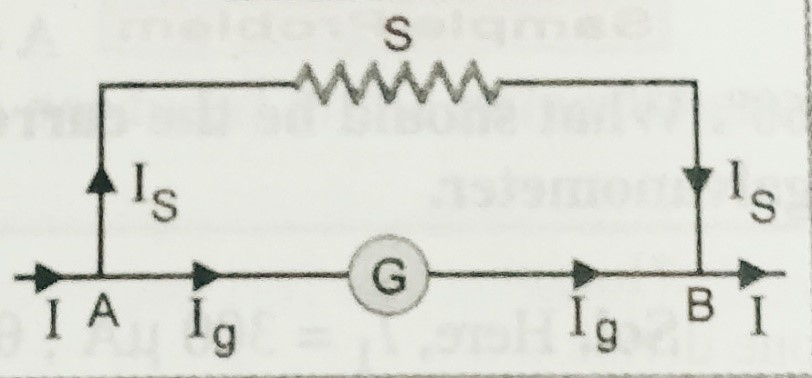Categories

# Shunt

In this topic we will discuss about, what is shunt? what are the uses of shunt? and how can we convert a galvanometer into ammeter?

Before to go with this topic students must know about the moving coil galvanometer, to get the notes of moving coil galvanometer click here-

Shunt-

Shunt is a low resistance connected in parallel with the galvanometer or ammeter to protect it from high current. It is used to convert the galvanometer into an ammeter, by connecting it in parallel.

When high value current passes through the galvanometer or a low range ammeter then it can damage the instrument . To overcome this difficulties a low value resistance(shunt) is connected in parallel , so that maximum current can pass through the shunt ,and instrument will remain  safe.

Let, I ,IS and IG are the total current passes through the circuit , galvanometer and circuit respectively. G , and S are the resistances of the galvanometer and shunt respectively.As shown in figure,  potential across S is VS = IS S

Potential across  G is  VG = IG G

Since both are in parallel combination , hence  is  VS = VG

So,  IS S =  IG G

But Is = I – IG

So we can write , ( I-IG ) S =  IG G ;and hence  IG = I (S /S+G)

Similarly , IS = I (G /S+G) ;

This result shows that shunt can be used as the (i) convert the galvanometer into ammeter and (ii) To increase the range of galvanometer.